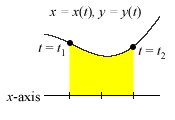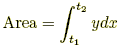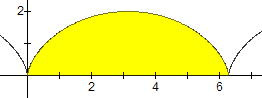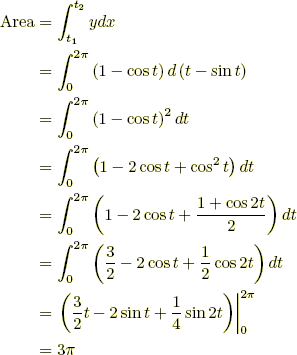index: click on a letter A B C D E F G H I J K L M N O P Q R S T U V W X Y Z A to Z index index: subject areas numbers & symbols sets, logic, proofs geometry algebra trigonometry advanced algebra & pre-calculus calculus advanced topics probability & statistics real world applications multimedia entrieswww.mathwords.com about mathwords website feedback

Area Using Parametric Equations
Parametric Integral Formula

The area between the x-axis and the graph of x = x(t), y = y(t) and the x-axis is given by the definite integral below. This formula gives a positive result for a graph above the x-axis, and a negative result for a graph below the x-axis.

Note: If the graph of x = x(t), y = y(t) is partly above and partly below the x-axis, the formula given below generates the net area. That is, the area above the axis minus the area below the axis.

 Formula:Example: Find the area of the between the x-axis and the first period of the cycloid x = t – sin t, y = 1 – cos t. The values of t run from 0 to 2π.